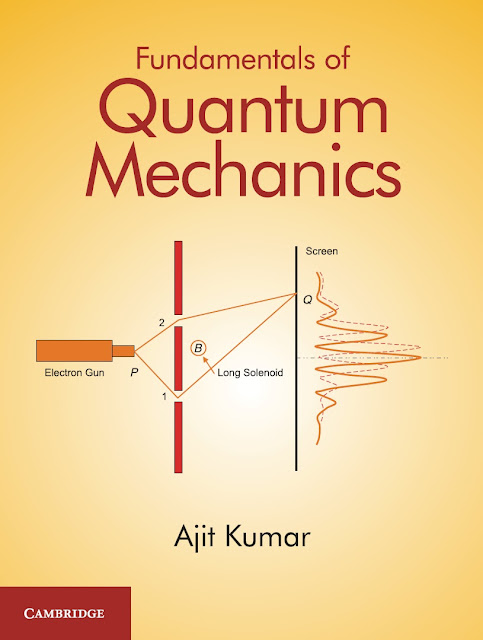# Fundamentals of Quantum Mechanics by Ajit KumarBook Fundamentals of Quantum Mechanics by Ajit Kumar is available to download free in pdf format.

 Name: Fundamentals of Quantum Mechanics by Ajit Kumar Format: pdf Categories: Chemistry Books,Physical Chemistry Publisher: Cambridge University Press

## About Fundamentals of Quantum Mechanics by Ajit Kumar

This book is a comprehensive text in the field of quantum mechanics, covering fundamental concepts including the state of a quantum mechanical system, operators, superposition principle and measurement postulate. The notion of an operator and the algebra of operators are introduced with the help of elementary concepts of mathematical analysis. Mathematical tools developed will help readers in understanding the difficulties encountered in classical physics while trying to explain the experimental results involving atomic spectra and other phenomena. The differential equations that arise while solving eigenvalue problems are solved rigorously, to make the text self-sufficient. The solutions are then physically interpreted and explained. The text offers solved examples, analogous and homework problems to help students in solving practical problems of physics requiring quantum mechanical treatment.